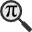## General

Display information for equation id:math.229921.11 on revision:229921

* Page found: Morera's theorem (eq math.229921.11)

(force rerendering)

Cannot find the equation data in the database. Fetching from revision text.

Occurrences on the following pages:

Hash: ae539dfcc999c28e25a0f3ae65c1de79

TeX (original user input):

\gamma


TeX (checked):

\gamma


### LaTeXML (experimental; uses MathML) rendering

MathML (480 B / 245 B) :${\displaystyle\gamma}$
<math xmlns="http://www.w3.org/1998/Math/MathML" id="p1.1.m1.1" class="ltx_Math" alttext="{\displaystyle\gamma}" display="inline">
<semantics id="p1.1.m1.1a">
<mi id="p1.1.m1.1.1" xref="p1.1.m1.1.1.cmml">γ</mi>
<annotation-xml encoding="MathML-Content" id="p1.1.m1.1b">
<ci id="p1.1.m1.1.1.cmml" xref="p1.1.m1.1.1">γ</ci>
</annotation-xml>
<annotation encoding="application/x-tex" id="p1.1.m1.1c">{\displaystyle\gamma}</annotation>
</semantics>
[/itex]


SVG (1.017 KB / 625 B) :

### MathML with SVG or PNG fallback (recommended for modern browsers and accessibility tools) rendering

SVG image empty. Force Re-Rendering

SVG (0 B / 8 B) :

PNG (0 B / 8 B) :

$\gamma$## Translations to Computer Algebra Systems

### Translation to Maple

In Maple: gamma

\gamma: Could be the Euler-Mascheroni constant.

But it is also a Greek letter. Be aware, that this program translated the letter as a normal Greek letter and not as a constant!

Use the DLMF-Macro \EulerConstant to translate \gamma as a constant.


### Translation to Mathematica

In Mathematica: \[Gamma]

\gamma: Could be the Euler-Mascheroni constant.

But it is also a Greek letter. Be aware, that this program translated the letter as a normal Greek letter and not as a constant!

Use the DLMF-Macro \EulerConstant to translate \gamma as a constant.


## Similar pages

Calculated based on the variables occurring on the entire Morera's theorem page

## Identifiers

• $\gamma$### MathML observations

0results

0results

no statistics present please run the maintenance script ExtractFeatures.php

0 results

0 results